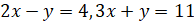APPLICATION OF LINEAR AND QUADRATIC EQUATION

Mathematics SSS 3 First Term

WEEK 5

APPLICATION OF LINEAR AND QUADRATIC EQUATION

Performance Objectives

Student should be able to:

1. Solve simultaneous linear and quadratic equations.
2. Solve word problem on simultaneous linear equations.
3. Solve word problem on simultaneous equations one linear one quadratic.

Content

Simultaneous Linear Equations

At this stage, we already have an idea or two on the steps taken to solve problems involving simultaneous linear equations hence, we go straight to examples to refresh our memory on the steps involved. Now simultaneous equations can be solved using either the substitution method or the elimination method.

Example 1: Solve the simultaneous equationusing

View More